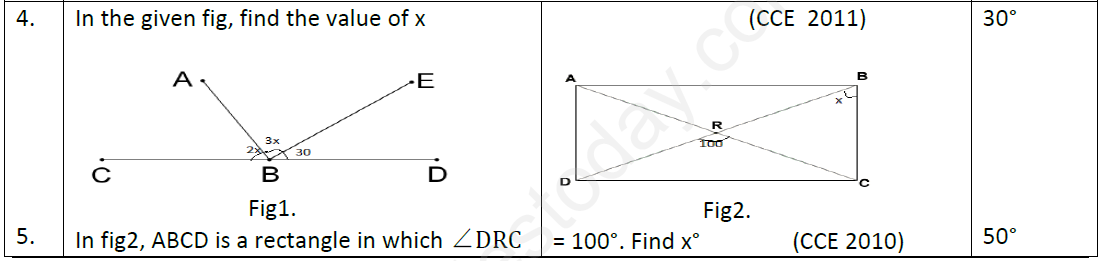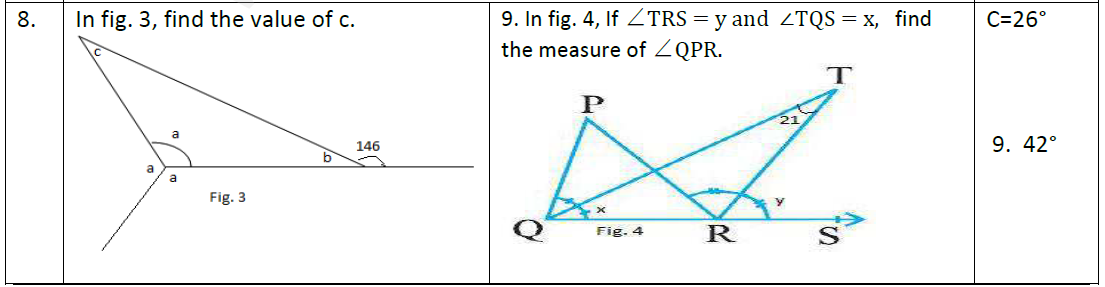# CBSE Class 9 Mathematics Lines and Angles Assignment Set B

Read and download free pdf of CBSE Class 9 Mathematics Lines and Angles Assignment Set B. Get printable school Assignments for Class 9 Mathematics. Standard 9 students should practise questions and answers given here for Chapter 6 Lines And Angles Mathematics in Grade 9 which will help them to strengthen their understanding of all important topics. Students should also download free pdf of Printable Worksheets for Class 9 Mathematics prepared as per the latest books and syllabus issued by NCERT, CBSE, KVS and do problems daily to score better marks in tests and examinations

## Assignment for Class 9 Mathematics Chapter 6 Lines And Angles

Class 9 Mathematics students should refer to the following printable assignment in Pdf for Chapter 6 Lines And Angles in standard 9. This test paper with questions and answers for Grade 9 Mathematics will be very useful for exams and help you to score good marks

### Chapter 6 Lines And Angles Class 9 Mathematics Assignment

SECTION B=A: (2 MARKS)

1. An angle is 20° more than three times the other angle. If the two angles are supplementary, find the angles. 40°, 140°

2. An exterior angle of a triangle is 105° and its two interior opposite angles are equal. Find each of these equal angles. 52 ½ °

3. If two times the measure of one angle is three times the other which is complement, find the angles. 36°, 54°SECTION B: (2 MARKS)

6. The exterior angles obtained on producing the base of a triangle both ways are 100° and 120°. Find all the angles  80°, 60°, 40°

7. In ΔABC, ∠A − ∠B = 63°, ∠B − ∠C = 18°.Find the measure of all the angles 108°, 45°, 27°9. In fig. 4, If ∠TRS = y and ∠TQS = x, find the measure of ∠QPR. C=26° 9. 42°

SECTION C: (3 MARKS)

10. 12. In fig.5, PS⊥ l and RQ ⊥l, find y° In the given figure , which of the

11. In fig.6 AC ǁ DE, ∠CBA = 28° find the value of x and ∠CAB. (HOTS) two lines are parallel and why? (NCERT EXEMPLAR)

10. 80° 11. 153°, 27° Yes, No

SECTION D: (4 MARKS)

13. The sides BC, CA and AB of a ΔABC are produced in order, forming exterior angles ∠ACD, ∠BAE and ∠CBF. Show that ∠ACD + ∠BAE + ∠CBF = 360°

14. If the bisectors of the angles B and C of ΔABC meet at a point O, then prove that ∠BOC =90° + ½ ∠A

15. In the given figure, lines PQ and RS intersect at O. If ∠POT = 75°, find a, b and c. 84°, 21°, 48°